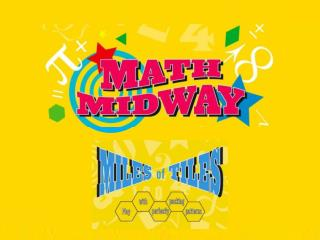DownloadDownload PresentationMiles of Tiles

# Miles of Tiles

Télécharger la présentation## Miles of Tiles

- - - - - - - - - - - - - - - - - - - - - - - - - - - E N D - - - - - - - - - - - - - - - - - - - - - - - - - - -
##### Presentation Transcript

1. Miles of Tiles The Exhibit

2. Miles of Tiles The Exhibit

3. Miles of Tiles The Exhibit

4. Miles of Tiles The Exhibit

5. Miles of Tiles The Exhibit

6. Math Geometry + Nature

7. Math Geometry + Nature Why do bees use hexagons? Is there something special about them?

8. Math Geometry + Nature Let’s compare what we calculated P= 12 A = 10.38 P= 12 A = 9 P= 12 A = 11.47

9. Math Geometry + Nature A circle yields the greatest interior space for the smallest perimeter of anyshape! P= 12 A = 11.47

10. Math Geometry + Nature Hexagons yields the greatest interior space for the smallest perimeter of any shape that tessellates! P= 12 A = 10.38

12. Lesson Ideas Grade 3 Develop Understanding of fractions as numbers Fraction  Fracture

13. Lesson Ideas Grade 3 Develop Understanding of fractions as numbers Fracture - break into pieces

14. Lesson Ideas Grade 3 Develop Understanding of fractions as numbers Fractions - break into pieces using numbers

15. Lesson Ideas Grade 3 Develop Understanding of fractions as numbers 4 = 1 1 1 1

16. Lesson Ideas Grade 3 Develop Understanding of fractions as numbers 4 = 1 1 1 1¼ means you have one of the four 1s

17. Lesson Ideas Grade 3 Develop Understanding of fractions as numbers You have one of the four squares

18. Lesson Ideas Grade 3 Develop Understanding of fractions as numbers Tiles provide a concrete example of the abstract concept of fractions

19. Lesson Ideas Grade 3 Understand the concepts of area and relate it to multiplication and addition

22. Lesson Ideas Grade 3 Area relationship to multiplication & addition 1 1 1 1

23. Lesson Ideas Grade 3 Area relationship to multiplication & addition 2 2

24. Lesson Ideas Grade 3 Area relationship to multiplication & addition 2 2 2 x 2 = 4

25. Lesson Ideas Grade 3 Area relationship to multiplication & addition Area of this square is 4 2 x 2 = 4

27. Lesson Ideas Grade 4 Understanding fraction equivalence

28. Lesson Ideas Grade 4 Understanding fraction equivalence

29. Lesson Ideas Grade 4 Understanding fraction equivalence

30. Lesson Ideas Grade 4 Understanding fraction equivalence 4/16 = 1/4

31. Lesson Ideas Grade 4 Understanding decimal notation for fractions

32. Lesson Ideas Grade 4 Understanding decimal notation for fractions ¼ spoken as “one quarter”

33. Lesson Ideas Grade 4 Understanding decimal notation for fractions With money ¼ of a dollar is one quarter

34. Lesson Ideas Grade 4 Understanding decimal notation for fractions One quarter is twenty-five cents 25¢

35. Lesson Ideas Grade 4 Understanding decimal notation for fractions Twenty-five cents is written as 25¢ .25

36. Lesson Ideas Grade 4 Understanding decimal notation for fractions .25 .25 .25 .25 \$1

37. Lesson Ideas Grade 5 Understanding concepts of volume

39. Lesson Ideas Grade 5 Understanding concepts of volume Stack the physical shapes on the board

40. Lesson Ideas Grade 5 Understanding concepts of volume Explain that the volume for all simple shapes is area x height

41. Lesson Ideas Grade 5 Understanding concepts of volume Explain that the volume for all simple shapes is area x height

42. Lesson Ideas Grade 5 Understanding concepts of volume Explain that the volume for all simple shapes is area x height

43. Lesson Ideas Grade 5 Understanding concepts of volume Explain that the volume for all simple shapes is area x height

44. Lesson Ideas Grade 5 Understanding concepts of volume Compare volumes to illustrate the bee’s use of hexagons

45. Lesson Ideas Grade 6 Solve real-world problems involving area

46. Lesson Ideas Grade 6 Solve real-world problems involving area You are installing new tiling on your bathroom floor

47. Lesson Ideas Grade 6 Solve real-world problems involving area

48. Lesson Ideas Grade 6 Solve real-world problems involving area